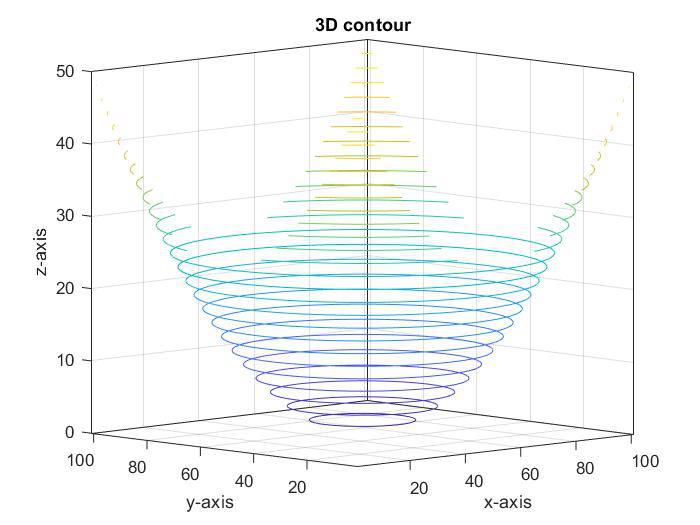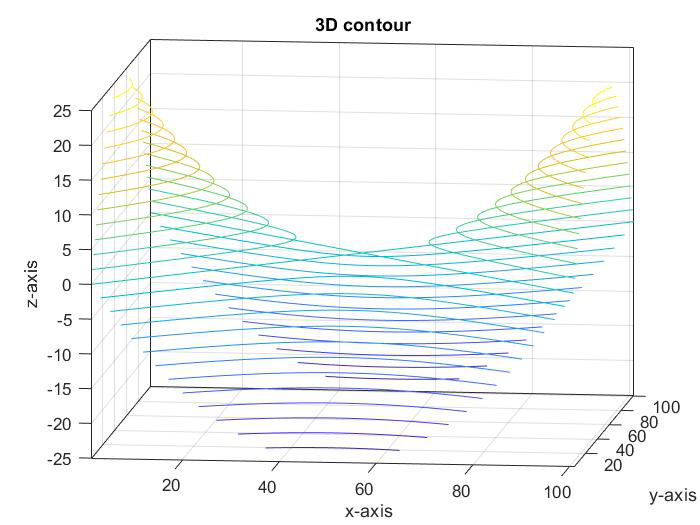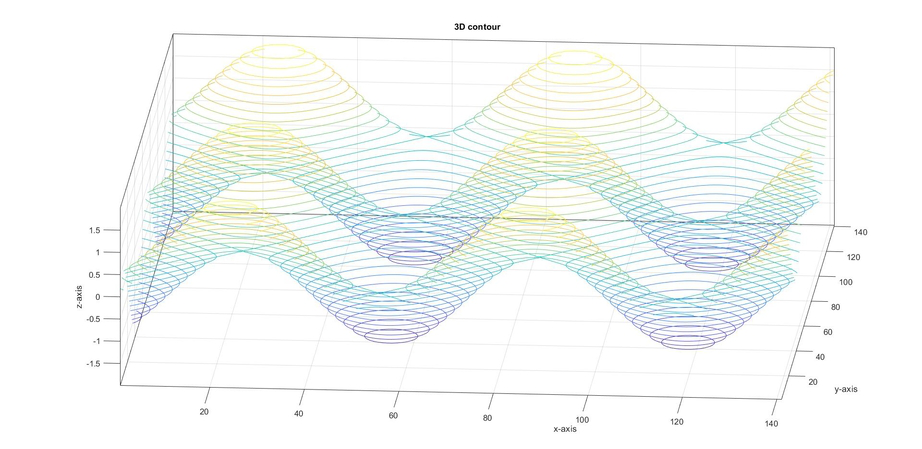Related Articles

# Plot a 3D Contour in MATLAB

• Difficulty Level : Medium
• Last Updated : 21 Apr, 2021

Contour plots are used to show 3D surfaces by plotting z- slides on a 2D surface. A contour plot is also called a line plot. In contour, we have 3 variables x, y, z. The x, y variables are used to give the values for z, ( z=f(x, y)). The x and y variables are usually in a grid called meshgrid.

There are various contour plots in MATLAB like contour, contourf, contour3, contourc, countourslice, clabel, and fcontour. In this article, we will see how to plot 3D contour in MATLAB. To plot 3D contour we will use countour3() to plot different types of 3D modules.

Syntax:

• contour3(X,Y,Z): Specifies the x and y coordinates for the values in Z.
• contour3(Z): Creates a 3-D contour plot containing the isolines of matrix Z, where Z contains height values on the x-y plane.
• contour3(___,levels): Specify levels as a scalar value n to display the contour lines at n automatically chosen levels (heights).
• contour3(___,LineSpec): Specifies the style and color of the contour line.

Step-wise Approach:

• Enter the inputs for x and y in meshgrid function.

## Matlab

 `% plot the points for x and y``[x,y]=meshgrid(-5 : 0.1 :5);`
• Pass the value for z using x and y in an equation.

## Matlab

 `% give any equation for z variable``z = x.^2 + y.^2 ;`` ` `% disp is used to display the ``% value for z``disp(z);`
• Use contour3 to plot 3d contour and to label the axis use label() method.

## Matlab

 `% to plot the 3D surface use contour3()``% contour3(z ,levels), levels is used to ``% display the height for the contourlines``contour3(z,25);`` ` `% to label x ,y ,z axis and title.``xlabel(``'x-axis'``);``ylabel(``'y-axis'``);``zlabel(``'z-axis'``);``title(``'3D contour'``);`

Below is the complete program:

## Matlab

 `% code for 3D plot``% plot the points for x and y``[x,y]=meshgrid(-5 : 0.1 :5);`` ` `% give any equation for z variable``z = x.^2 + y.^2 ;``disp(z);`` ` `% to plot the 3D surface use contour3()``% contour3(z ,levels), levels is used ``% to display the height for the contour``% lines``contour3(z,25);`` ` `% to label x ,y ,z axis and title.``xlabel(``'x-axis'``);``ylabel(``'y-axis'``);``zlabel(``'z-axis'``);``title(``'3D contour'``);`

Output:Example 1:

Here is another example similar to the previous one.

## Matlab

 `% code for 3D plot,``% plot the points for x and y``[x,y]=meshgrid(-5 : 0.1 :5);`` ` `% give any equation for z variable``z = x.^2 - y.^2 ;``disp(z);`` ` `% to plot the 3D surface use contour3()``% contour3(z ,levels), levels is used to``% display the height for the contourlines``contour3(z,25);`` ` `% to label x ,y , z axis and title``xlabel(``'x-axis'``);``ylabel(``'y-axis'``);``zlabel(``'z-axis'``);``title(``'3D contour'``);`

Output:Example 2:

MATLAB program to generate a 3D Contour:

## Matlab

 `% code for 3D plot,``% plot the points for x and y``[x,y]=meshgrid(-7 : 0.1 : 7);`` ` `% give any equation for z variable``z = sin(x)+ cos(y) ;``disp(z);`` ` `% to plot the 3D surface use contour3()``% contour3(z ,levels), levels is used to ``% display the height for the contour``% lines``contour3(z,25);`` ` `% to label x ,y and z axis``xlabel(``'x-axis'``);``ylabel(``'y-axis'``);``zlabel(``'z-axis'``);``title(``'3D contour'``);`

Output:My Personal Notes arrow_drop_up